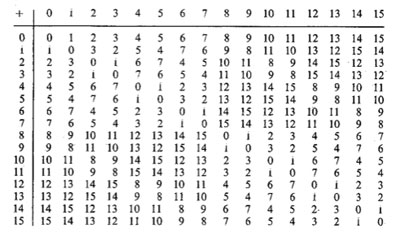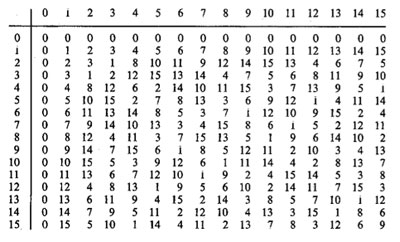Last time we did recall Cantor’s addition and multiplication on ordinal numbers. Note that we can identify an ordinal number $\alpha$ with (the order type of) the set of all strictly smaller ordinals, that is, $\alpha = { \alpha’~:~\alpha’ < \alpha }$. Given two ordinals $\alpha$ and $\beta$ we will denote their Cantor-sums and products as $[ \alpha + \beta]$ and $[\alpha . \beta]$.

The reason for these square brackets is that John Conway constructed a well behaved nim-addition and nim-multiplication on all ordinals $\mathbf{On}_2$ by imposing the ‘simplest’ rules which make $\mathbf{On}_2$ into a field. By this we mean that, in order to define the addition $\alpha + \beta$ we must have constructed before all sums $\alpha’ + \beta$ and $\alpha + \beta’$ with $\alpha’ < \alpha$ and $\beta’ < \beta$. If + is going to be a well-defined addition on $\mathbf{On}_2$ clearly $\alpha + \beta$ cannot be equal to one of these previously constructed sums and the ‘simplicity rule’ asserts that we should take $\alpha+\beta$ the least ordinal different from all these sums $\alpha’+\beta$ and $\alpha+\beta’$. In symbols, we define

$\alpha+ \beta = \mathbf{mex} { \alpha’+\beta,\alpha+ \beta’~|~\alpha’ < \alpha, \beta’ < \beta }$

where $\mathbf{mex}$ stands for ‘minimal excluded value’. If you’d ever played the game of Nim you will recognize this as the Nim-addition, at least when $\alpha$ and $\beta$ are finite ordinals (that is, natural numbers) (to nim-add two numbers n and m write them out in binary digits and add without carrying). Alternatively, the nim-sum n+m can be found applying the following two rules :

• the nim-sum of a number of distinct 2-powers is their ordinary sum (e.g. $8+4+1=13$, and,
• the nim-sum of two equal numbers is 0.

So, all we have to do is to write numbers n and m as sums of two powers, scratch equal terms and add normally. For example, $13+7=(8+4+1)+(4+2+1)=8+2=10$ (of course this is just digital sum without carry in disguise).Here’s the beginning of the nim-addition table on ordinals. For example, to define $13+7$ we have to look at all values in the first 7 entries of the row of 13 (that is, ${ 13,12,15,14,9,8,11 }$) and the first 13 entries in the column of 7 (that is, ${ 7,6,5,4,3,2,1,0,15,14,13,12,11 }$) and find the first number not included in these two sets (which is indeed $10$).

In fact, the above two rules allow us to compute the nim-sum of any two ordinals. Recall from last time that every ordinal can be written uniquely as as a finite sum of (ordinal) 2-powers :
$\alpha = [2^{\alpha_0} + 2^{\alpha_1} + \ldots + 2^{\alpha_k}]$, so to determine the nim-sum $\alpha+\beta$ we write both ordinals as sums of ordinal 2-powers, delete powers appearing twice and take the Cantor ordinal sum of the remaining sum.Nim-multiplication of ordinals is a bit more complicated. Here’s the definition as a minimal excluded value

$\alpha.\beta = \mathbf{mex} { \alpha’.\beta + \alpha.\beta’ – \alpha’.\beta’ }$

for all $\alpha’ < \alpha, \beta’ < \beta$. The rationale behind this being that both $\alpha-\alpha’$ and $\beta – \beta’$ are non-zero elements, so if $\mathbf{On}_2$ is going to be a field under nim-multiplication, their product should be non-zero (and hence strictly greater than 0), that is, $~(\alpha-\alpha’).(\beta-\beta’) > 0$. Rewriting this we get $\alpha.\beta > \alpha’.\beta+\alpha.\beta’-\alpha’.\beta’$ and again the ‘simplicity rule’ asserts that $\alpha.\beta$ should be the least ordinal satisfying all these inequalities, leading to the $\mathbf{mex}$-definition above. The table gives the beginning of the nim-multiplication table for ordinals. For finite ordinals n and m there is a simple 2 line procedure to compute their nim-product, similar to the addition-rules mentioned before :

• the nim-product of a number of distinct Fermat 2-powers (that is, numbers of the form $2^{2^n}$) is their ordinary product (for example, $16.4.2=128$), and,
• the square of a Fermat 2-power is its sesquimultiple (that is, the number obtained by multiplying with $1\frac{1}{2}$ in the ordinary sense). That is, $2^2=3,4^2=6,16^2=24,…$

Using these rules, associativity and distributivity and our addition rules it is now easy to work out the nim-multiplication $n.m$ : write out n and m as sums of (multiplications by 2-powers) of Fermat 2-powers and apply the rules. Here’s an example

$5.9=(4+1).(4.2+1)=4^2.2+4.2+4+1=6.2+8+4+1=(4+2).2+13=4.2+2^2+13=8+3+13=6$

Clearly, we’d love to have a similar procedure to calculate the nim-product $\alpha.\beta$ of arbitrary ordinals, or at least those smaller than $\omega^{\omega^{\omega}}$ (recall that Conway proved that this ordinal is isomorphic to the algebraic closure $\overline{\mathbb{F}}_2$ of the field of two elements). From now on we restrict to such ‘small’ ordinals and we introduce the following special elements :

$\kappa_{2^n} = [2^{2^{n-1}}]$ (these are the Fermat 2-powers) and for all primes $p > 2$ we define
$\kappa_{p^n} = [\omega^{\omega^{k-1}.p^{n-1}}]$ where $k$ is the number of primes strictly smaller than $p$ (that is, for p=3 we have k=1, for p=5, k=2 etc.).

Again by associativity and distributivity we will be able to multiply two ordinals $< \omega^{\omega^{\omega}}$ if we know how to multiply a product

$[\omega^{\alpha}.2^{n_0}].[\omega^{\beta}.2^{m_0}]$ with $\alpha,\beta < [\omega^{\omega}]$ and $n_0,m_0 \in \mathbb{N}$.

Now, $\alpha$ can be written uniquely as $[\omega^t.n_t+\omega^{t-1}.n_{t-1}+\ldots+\omega.n_2 + n_1]$ with t and all $n_i$ natural numbers. Write each $n_k$ in base $p$ where $p$ is the $k+1$-th prime number, that is, we have for $n_0,n_1,\ldots,n_t$ an expression

$n_k=[\sum_j p^j.m(j,k)]$ with $0 \leq m(j,k) < p$

The point of all this is that any of the special elements we want to multiply can be written as a unique expression as a decreasing product

$[\omega^{\alpha}.2^{n_0}] = [ \prod_q \kappa_q^m(q) ]$

where $q$ runs over all prime powers. The crucial fact now is that for this decreasing product we have a rule similar to addition of 2-powers, that is Conway-products coincide with the Cantor-products

$[ \prod_q \kappa_q^m(q) ] = \prod_q \kappa_q^m(q)$

But then, using associativity and commutativity of the Conway-product we can ‘nearly’ describe all products $[\omega^{\alpha}.2^{n_0}].[\omega^{\beta}.2^{m_0}]$. The remaining problem being that it may happen that for some q we will end up with an exponent $m(q)+m(q’)>p$. But this can be solved if we know how to take p-powers. The rules for this are as follows

$~(\kappa_{2^n})^2 = \kappa_{2^n} + \prod_{1 \leq i < n} \kappa_{2^i}$, for 2-powers, and,

$~(\kappa_{p^n})^p = \kappa_{p^{n-1}}$ for a prime $p > 2$ and for $n \geq 2$, and finally

$~(\kappa_p)^p = \alpha_p$ for a prime $p > 2$, where $\alpha_p$ is the smallest ordinal $< \kappa_p$ which cannot be written as a p-power $\beta^p$ with $\beta < \kappa_p$. Summarizing : if we will be able to find these mysterious elements $\alpha_p$ for all prime numbers p, we are able to multiply in $[\omega^{\omega^{\omega}}]=\overline{\mathbb{F}}_2$.

Let us determine the first one. We have that $\kappa_3 = \omega$ so we are looking for the smallest natural number $n < \omega$ which cannot be written in num-multiplication as $n=m^3$ for $m < \omega$ (that is, also $m$ a natural number). Clearly $1=1^3$ but what about 2? Can 2 be a third root of a natural number wrt. nim-multiplication? From the tabel above we see that 2 has order 3 whence its cube root must be an element of order 9. Now, the only finite ordinals that are subfields of $\mathbf{On}_2$ are precisely the Fermat 2-powers, so if there is a finite cube root of 2, it must be contained in one of the finite fields $[2^{2^n}]$ (of which the mutiplicative group has order $2^{2^n}-1$ and one easily shows that 9 cannot be a divisor of any of the numbers $2^{2^n}-1$, that is, 2 doesn’t have a finte 3-th root in nim! Phrased differently, we found our first mystery number $\alpha_3 = 2$. That is, we have the marvelous identity in nim-arithmetic

$\omega^3 = 2$

Okay, so what is $\alpha_5$? Well, we have $\kappa_5 = [\omega^{\omega}]$ and we have to look for the smallest ordinal which cannot be written as a 5-th root. By inspection of the finite nim-table we see that 1,2 and 3 have 5-th roots in $\omega$ but 4 does not! The reason being that 4 has order 15 (check in the finite field ) and 25 cannot divide any number of the form $2^{2^n}-1$. That is, $\alpha_5=4$ giving another crazy nim-identity

$~(\omega^{\omega})^5 = 4$

And, surprises continue to pop up… Conway showed that $\alpha_7 = \omega+1$ giving the nim-identity $~(\omega^{\omega^2})^7 = \omega+1$. The proof of this already uses some clever finite field arguments. Because 7 doesn’t divide any number $2^{2^n}-1$, none of the finite subfields $[2^{2^n}]$ contains a 7-th root of unity, so the 7-power map is injective whence surjective, so all finite ordinal have finite 7-th roots! That is, $\alpha_7 \geq \omega$. Because $\omega$ lies in a cubic extension of the finite field , the field generated by $\omega$ has 64 elements and so its multiplicative group is cyclic of order 63 and as $\omega$ has order 9, it must be a 7-th power in this field. But, as the only 7th powers in that field are precisely the powers of $\omega$ and by inspection $\omega+1$ is not a 7-th power in that field (and hence also not in any field extension obtained by adjoining square, cube and fifth roots) so $\alpha_7=\omega +1$.

Conway did stop at $\alpha_7$ but I’ve always been intrigued by that one line in ONAG p.61 : “Hendrik Lenstra has computed $\alpha_p$ for $p \leq 43$”. Next time we will see how Lenstra managed to do this and we will use sage to extend his list a bit further, including the first open case : $\alpha_{47}= \omega^{\omega^7}+1$.

For an enjoyable video on all of this, see Conway’s MSRI lecture on Infinite Games. The nim-arithmetic part is towards the end of the lecture but watching the whole video is a genuine treat!

Published in featured games number theory